## DengAI: Predicting Disease Spread - Benchmark

###### Fri 23 December 2016 by Peter Bull

Dengue fever is bad. It's real bad. Dengue is a mosquito-borne disease that occurs in tropical and sub-tropical parts of the world. In mild cases, symptoms are similar to the flu: fever, rash and muscle and joint pain. But severe cases are dangerous, and dengue fever can cause severe bleeding, low blood pressure and even death.

Because it is carried by mosquitoes, the transmission dynamics of dengue are related to climate variables such as temperature and precipitation. Although the relationship to climate is complex, a growing number of scientists argue that climate change is likely to produce distributional shifts that will have significant public health implications worldwide.

We've launched a competition to use open data to predict the occurrence of Dengue based on climatological data. Here's a first look at the data and how to get started!

As always, we begin with the sacred import's of data science:

In :
%matplotlib inline

from __future__ import print_function
from __future__ import division

import pandas as pd
import numpy as np

from matplotlib import pyplot as plt
import seaborn as sns

from sklearn.model_selection import train_test_split
import statsmodels.api as sm

# just for the sake of this blog post!
from warnings import filterwarnings
filterwarnings('ignore')


## A Tale of Two Cities¶This dataset has two cities in it: San Juan, Puerto Rico (right) and Iquitos, Peru (left). Since we hypothesize that the spread of dengue may follow different patterns between the two, we will divide the dataset, train seperate models for each city, and then join our predictions before making our final submission.

In :
# load the provided data
index_col=[0,1,2])

index_col=[0,1,2])

In :
# Seperate data for San Juan
sj_train_features = train_features.loc['sj']
sj_train_labels = train_labels.loc['sj']

# Separate data for Iquitos
iq_train_features = train_features.loc['iq']
iq_train_labels = train_labels.loc['iq']

In :
print('San Juan')
print('features: ', sj_train_features.shape)
print('labels  : ', sj_train_labels.shape)

print('\nIquitos')
print('features: ', iq_train_features.shape)
print('labels  : ', iq_train_labels.shape)

San Juan
features:  (936, 21)
labels  :  (936, 1)

Iquitos
features:  (520, 21)
labels  :  (520, 1)


The problem description gives a good overview of the available variables, but we'll look at the head of the data here as well:

In :
sj_train_features.head()

Out:
week_start_date ndvi_ne ndvi_nw ndvi_se ndvi_sw precipitation_amt_mm reanalysis_air_temp_k reanalysis_avg_temp_k reanalysis_dew_point_temp_k reanalysis_max_air_temp_k ... reanalysis_precip_amt_kg_per_m2 reanalysis_relative_humidity_percent reanalysis_sat_precip_amt_mm reanalysis_specific_humidity_g_per_kg reanalysis_tdtr_k station_avg_temp_c station_diur_temp_rng_c station_max_temp_c station_min_temp_c station_precip_mm
year weekofyear
1990 18 1990-04-30 0.122600 0.103725 0.198483 0.177617 12.42 297.572857 297.742857 292.414286 299.8 ... 32.00 73.365714 12.42 14.012857 2.628571 25.442857 6.900000 29.4 20.0 16.0
19 1990-05-07 0.169900 0.142175 0.162357 0.155486 22.82 298.211429 298.442857 293.951429 300.9 ... 17.94 77.368571 22.82 15.372857 2.371429 26.714286 6.371429 31.7 22.2 8.6
20 1990-05-14 0.032250 0.172967 0.157200 0.170843 34.54 298.781429 298.878571 295.434286 300.5 ... 26.10 82.052857 34.54 16.848571 2.300000 26.714286 6.485714 32.2 22.8 41.4
21 1990-05-21 0.128633 0.245067 0.227557 0.235886 15.36 298.987143 299.228571 295.310000 301.4 ... 13.90 80.337143 15.36 16.672857 2.428571 27.471429 6.771429 33.3 23.3 4.0
22 1990-05-28 0.196200 0.262200 0.251200 0.247340 7.52 299.518571 299.664286 295.821429 301.9 ... 12.20 80.460000 7.52 17.210000 3.014286 28.942857 9.371429 35.0 23.9 5.8

5 rows × 21 columns

There are a lot of climate variables here, but the first thing that we'll note is that the week_start_date is included in the feature set. This makes it easier for competitors to create time based features, but for this first-pass model, we'll drop that column since we shouldn't use it as a feature in our model.

In :
# Remove week_start_date string.
sj_train_features.drop('week_start_date', axis=1, inplace=True)
iq_train_features.drop('week_start_date', axis=1, inplace=True)


Next, let's check to see if we are missing any values in this dataset:

In :
# Null check
pd.isnull(sj_train_features).any()

Out:
ndvi_ne                                  True
ndvi_nw                                  True
ndvi_se                                  True
ndvi_sw                                  True
precipitation_amt_mm                     True
reanalysis_air_temp_k                    True
reanalysis_avg_temp_k                    True
reanalysis_dew_point_temp_k              True
reanalysis_max_air_temp_k                True
reanalysis_min_air_temp_k                True
reanalysis_precip_amt_kg_per_m2          True
reanalysis_relative_humidity_percent     True
reanalysis_sat_precip_amt_mm             True
reanalysis_specific_humidity_g_per_kg    True
reanalysis_tdtr_k                        True
station_avg_temp_c                       True
station_diur_temp_rng_c                  True
station_max_temp_c                       True
station_min_temp_c                       True
station_precip_mm                        True
dtype: bool
In :
(sj_train_features
.ndvi_ne
.plot
.line(lw=0.8))

plt.title('Vegetation Index over Time')
plt.xlabel('Time')

Out:
<matplotlib.text.Text at 0x117d0d898>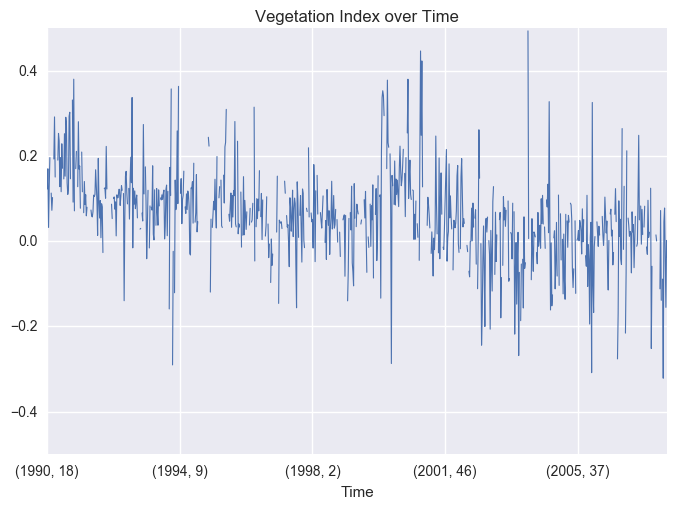Since these are time-series, we can see the gaps where there are NaNs by plotting the data. Since we can't build a model without those values, we'll take a simple approach and just fill those values with the most recent value that we saw up to that point. This is probably a good part of the problem to improve your score by getting smarter.

In :
sj_train_features.fillna(method='ffill', inplace=True)
iq_train_features.fillna(method='ffill', inplace=True)


## Distribution of labels¶

Our target variable, total_cases is a non-negative integer, which means we're looking to make some count predictions. Standard regression techniques for this type of prediction include

1. Poisson regression
2. Negative binomial regression

Which techniqe will perform better depends on many things, but the choice between Poisson regression and negative binomial regression is pretty straightforward. Poisson regression fits according to the assumption that the mean and variance of the population distributiona are equal. When they aren't, specifically when the variance is much larger than the mean, the negative binomial approach is better. Why? It isn't magic. The negative binomial regression simply lifts the assumption that the population mean and variance are equal, allowing for a larger class of possible models. In fact, from this perspective, the Poisson distribution is but a special case of the negative binomial distribution.

Let's see how our labels are distributed!

In :
print('San Juan')
print('mean: ', sj_train_labels.mean())
print('var :', sj_train_labels.var())

print('\nIquitos')
print('mean: ', iq_train_labels.mean())
print('var :', iq_train_labels.var())

San Juan
mean:  34.1805555556
var : 2640.04543969

Iquitos
mean:  7.56538461538
var : 115.895523937


It's looking like a negative-binomial sort of day in these parts.

In :
sj_train_labels.hist()

Out:
array([[<matplotlib.axes._subplots.AxesSubplot object at 0x117cff550>]], dtype=object)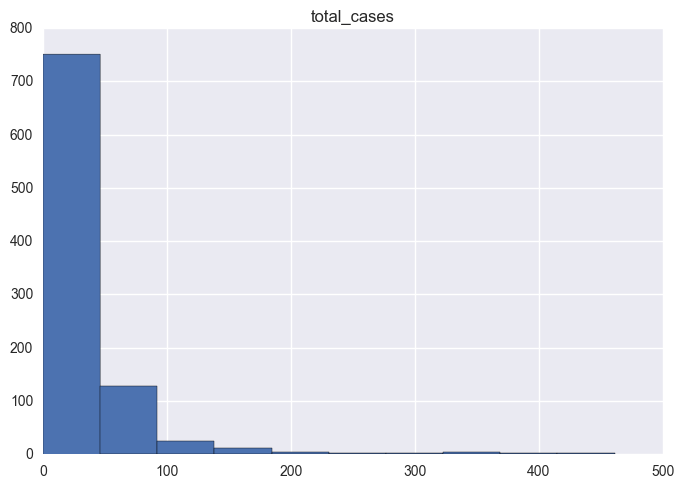In :
iq_train_labels.hist()

Out:
array([[<matplotlib.axes._subplots.AxesSubplot object at 0x11ace9208>]], dtype=object)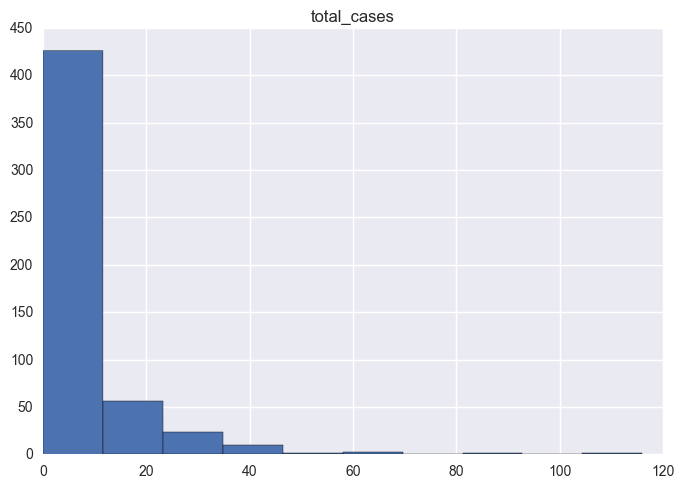## Which inputs strongly correlate with total_cases?¶

Our next step in this process will be to select a subset of features to include in our regression. Our primary purpose here is to get a better understanding of the problem domain rather than eke out the last possible bit of predictive accuracy. The first thing we will do is to add the total_cases to our dataframe, and then look at the correlation of that variable with the climate variables.

In :
sj_train_features['total_cases'] = sj_train_labels.total_cases
iq_train_features['total_cases'] = iq_train_labels.total_cases


Compute the data correlation matrix.

In :
# compute the correlations
sj_correlations = sj_train_features.corr()
iq_correlations = iq_train_features.corr()

In :
# plot san juan
sj_corr_heat = sns.heatmap(sj_correlations)
plt.title('San Juan Variable Correlations')

Out:
<matplotlib.text.Text at 0x11adac940>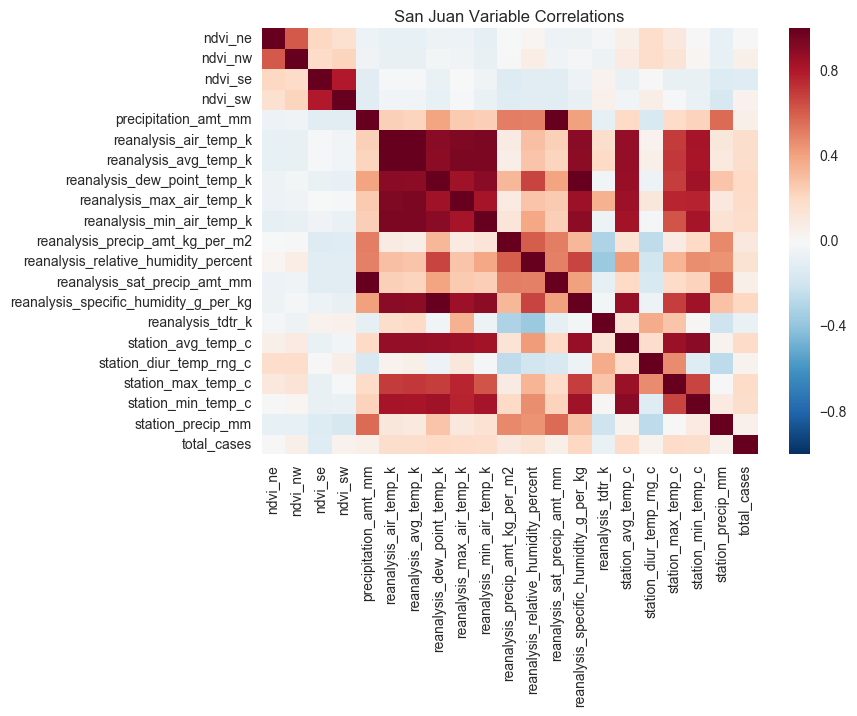In :
# plot iquitos
iq_corr_heat = sns.heatmap(iq_correlations)
plt.title('Iquitos Variable Correlations')

Out:
<matplotlib.text.Text at 0x11b360400>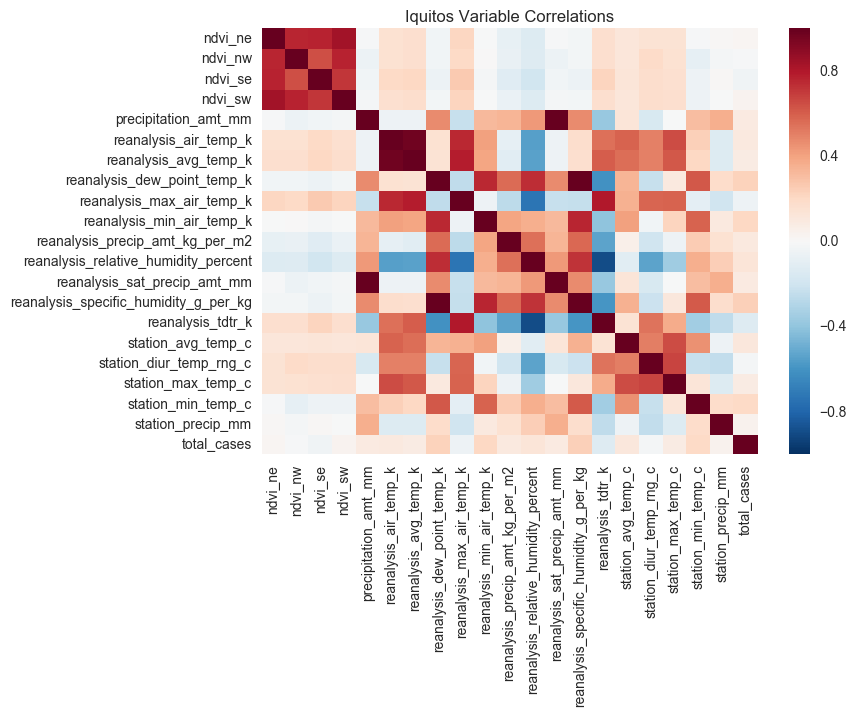### Many of the temperature data are strongly correlated, which is expected. But the total_cases variable doesn't have many obvious strong correlations.¶

Interestingly, total_cases seems to only have weak correlations with other variables. Many of the climate variables are much more strongly correlated. Interestingly, the vegetation index also only has weak correlation with other variables. These correlations may give us some hints as to how to improve our model that we'll talk about later in this post. For now, let's take a sorted look at total_cases correlations.

In :
# San Juan
(sj_correlations
.total_cases
.drop('total_cases') # don't compare with myself
.sort_values(ascending=False)
.plot
.barh())

Out:
<matplotlib.axes._subplots.AxesSubplot at 0x11b5f0978>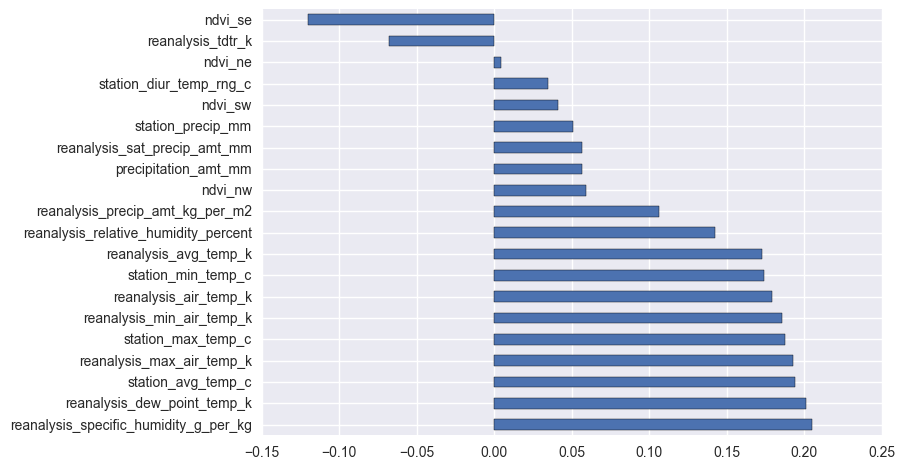In :
# Iquitos
(iq_correlations
.total_cases
.drop('total_cases') # don't compare with myself
.sort_values(ascending=False)
.plot
.barh())

Out:
<matplotlib.axes._subplots.AxesSubplot at 0x11b6c2d68>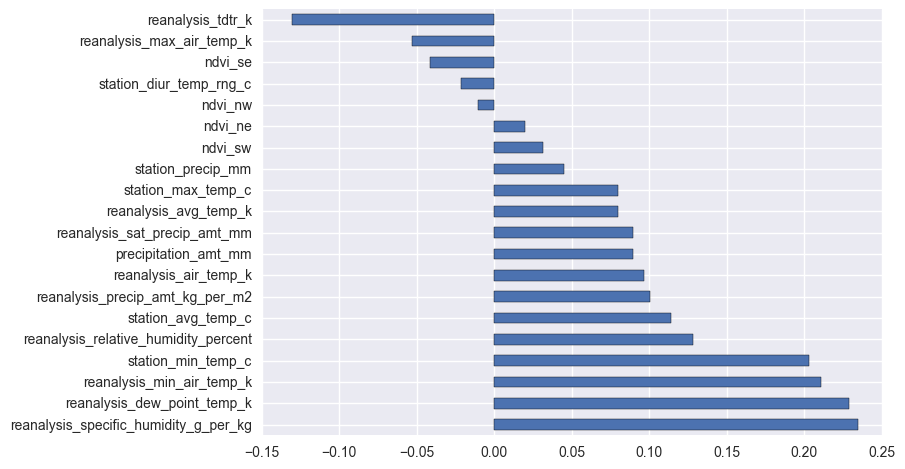### A few observations¶

#### The wetter the better¶

• The correlation strengths differ for each city, but it looks like reanalysis_specific_humidity_g_per_kg and reanalysis_dew_point_temp_k are the most strongly correlated with total_cases. This makes sense: we know mosquitos thrive wet climates, the wetter the better!

#### Hot and heavy¶

• As we all know, "cold and humid" is not a thing. So it's not surprising that as minimum temperatures, maximum temperatures, and average temperatures rise, the total_cases of dengue fever tend to rise as well.

#### Sometimes it rains, so what¶

• Interestingly, the precipitation measurements bear little to no correlation to total_cases, despite strong correlations to the humidity measurements, as evident by the heatmaps above.

### This is just a first pass¶

Precisely none of these correlations are very strong. Of course, that doesn't mean that some feature engineering wizardry can't put us in a better place (standing_water estimate, anyone?). Also, it's always useful to keep in mind that life isn't linear, but out-of-the-box correlation measurement is – or at least, it measures linear dependence.

Nevertheless, for this benchmark we'll focus on the linear wetness trend we see above, and reduce our inputs to

#### A few good variables¶

• reanalysis_specific_humidity_g_per_kg
• reanalysis_dew_point_temp_k
• station_avg_temp_c
• station_min_temp_c

## A mosquito model¶

Now that we've explored this data, it's time to start modeling. Our first step will be to build a function that does all of the preprocessing we've done above from start to finish. This will make our lives easier, since it needs to be applied to the test set and the traning set before we make our predictions.

In :
def preprocess_data(data_path, labels_path=None):
# load data and set index to city, year, weekofyear
df = pd.read_csv(data_path, index_col=[0, 1, 2])

# select features we want
features = ['reanalysis_specific_humidity_g_per_kg',
'reanalysis_dew_point_temp_k',
'station_avg_temp_c',
'station_min_temp_c']
df = df[features]

# fill missing values
df.fillna(method='ffill', inplace=True)

if labels_path:
labels = pd.read_csv(labels_path, index_col=[0, 1, 2])
df = df.join(labels)

# separate san juan and iquitos
sj = df.loc['sj']
iq = df.loc['iq']

return sj, iq

In :
sj_train, iq_train = preprocess_data('../data-processed/dengue_features_train.csv',
labels_path="../data-processed/dengue_labels_train.csv")


Now we can take a look at the smaller dataset and see that it's ready to start modelling:

In :
sj_train.describe()

Out:
reanalysis_specific_humidity_g_per_kg reanalysis_dew_point_temp_k station_avg_temp_c station_min_temp_c total_cases
count 936.000000 936.000000 936.000000 936.000000 936.000000
mean 16.547535 295.104736 26.999191 22.594017 34.180556
std 1.560663 1.570075 1.415079 1.506281 51.381372
min 11.715714 289.642857 22.842857 17.800000 0.000000
25% 15.233571 293.843929 25.842857 21.700000 9.000000
50% 16.835000 295.451429 27.214286 22.800000 19.000000
75% 17.854286 296.415714 28.175000 23.900000 37.000000
max 19.440000 297.795714 30.071429 25.600000 461.000000
In :
iq_train.describe()

Out:
reanalysis_specific_humidity_g_per_kg reanalysis_dew_point_temp_k station_avg_temp_c station_min_temp_c total_cases
count 520.000000 520.000000 520.000000 520.000000 520.000000
mean 17.102019 295.498723 27.506331 21.210385 7.565385
std 1.443048 1.414360 0.908973 1.257734 10.765478
min 12.111429 290.088571 21.400000 14.700000 0.000000
25% 16.121429 294.596429 26.957500 20.600000 1.000000
50% 17.428571 295.852143 27.587500 21.400000 5.000000
75% 18.180357 296.557143 28.075000 22.000000 9.000000
max 20.461429 298.450000 30.800000 24.200000 116.000000

## Split it up!¶

Since this is a timeseries model, we'll use a strict-future holdout set when we are splitting our train set and our test set. We'll keep around three quarters of the original data for training and use the rest to test. We'll do this separately for our San Juan model and for our Iquitos model.

In :
sj_train_subtrain = sj_train.head(800)
sj_train_subtest = sj_train.tail(sj_train.shape - 800)

iq_train_subtest = iq_train.tail(iq_train.shape - 400)


## Training time¶

This is where we start getting down to business. As we noted above, we'll train a NegativeBinomial model, which is often used for count data where the mean and the variance are very different. In this function we have three steps. The first is to specify the functional form

In :
from statsmodels.tools import eval_measures
import statsmodels.formula.api as smf

def get_best_model(train, test):
# Step 1: specify the form of the model
model_formula = "total_cases ~ 1 + " \
"reanalysis_specific_humidity_g_per_kg + " \
"reanalysis_dew_point_temp_k + " \
"station_min_temp_c + " \
"station_avg_temp_c"

grid = 10 ** np.arange(-8, -3, dtype=np.float64)

best_alpha = []
best_score = 1000

# Step 2: Find the best hyper parameter, alpha
for alpha in grid:
model = smf.glm(formula=model_formula,
data=train,
family=sm.families.NegativeBinomial(alpha=alpha))

results = model.fit()
predictions = results.predict(test).astype(int)
score = eval_measures.meanabs(predictions, test.total_cases)

if score < best_score:
best_alpha = alpha
best_score = score

print('best alpha = ', best_alpha)
print('best score = ', best_score)

# Step 3: refit on entire dataset
full_dataset = pd.concat([train, test])
model = smf.glm(formula=model_formula,
data=full_dataset,
family=sm.families.NegativeBinomial(alpha=best_alpha))

fitted_model = model.fit()
return fitted_model

sj_best_model = get_best_model(sj_train_subtrain, sj_train_subtest)
iq_best_model = get_best_model(iq_train_subtrain, iq_train_subtest)

best alpha =  1e-08
best score =  22.0808823529
best alpha =  1e-08
best score =  6.46666666667

In :
figs, axes = plt.subplots(nrows=2, ncols=1)

# plot sj
sj_train['fitted'] = sj_best_model.fittedvalues
sj_train.fitted.plot(ax=axes, label="Predictions")
sj_train.total_cases.plot(ax=axes, label="Actual")

# plot iq
iq_train['fitted'] = iq_best_model.fittedvalues
iq_train.fitted.plot(ax=axes, label="Predictions")
iq_train.total_cases.plot(ax=axes, label="Actual")

plt.suptitle("Dengue Predicted Cases vs. Actual Cases")
plt.legend()

Out:
<matplotlib.legend.Legend at 0x11c526940>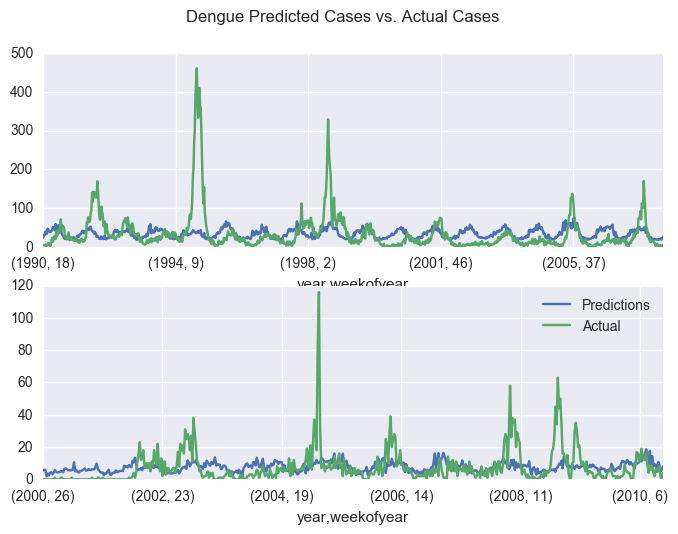## Reflecting on our performance¶

These graphs can actually tell us a lot about where our model is going wrong and give us some good hints about where investments will improve the model performance. For example, we see that our model in blue does track the seasonality of Dengue cases. However, the timing of the seasonality of our predictions has a mismatch with the actual results. One potential reason for this is that our features don't look far enough into the past--that is to say, we are asking to predict cases at the same time as we are measuring percipitation. Because dengue is misquito born, and the misquito lifecycle depends on water, we need to take both the life of a misquito and the time between infection and symptoms into account when modeling dengue. This is a critical avenue to explore when improving this model.

The other important error is that our predictions are relatively consistent--we miss the spikes that are large outbreaks. One reason is that we don't take into account the contagiousness of dengue. A possible way to account for this is to build a model that progressively predicts a new value while taking into account the previous prediction. By training on the dengue outbreaks and then using the predicted number of patients in the week before, we can start to model this time dependence that the current model misses.

So, we know we're not going to win this thing, but let's submit the model anyway!

In :
sj_test, iq_test = preprocess_data('../data-processed/dengue_features_test.csv')

sj_predictions = sj_best_model.predict(sj_test).astype(int)
iq_predictions = iq_best_model.predict(iq_test).astype(int)